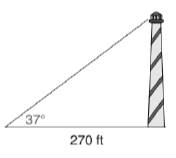Chapter 11.3, Problem 40EElementary Geometry For College St...

7th Edition
Alexander + 2 others
ISBN: 9781337614085

Solutions

Chapter
SectionElementary Geometry For College St...

7th Edition
Alexander + 2 others
ISBN: 9781337614085
Textbook Problem

In Exercises 39 to 45, angle measures should be given to the nearest degree; distance should be given to the nearest tenth of a unit.Kristine observes the top of a lookout tower from a point 270 ft from its base. If the indicated angle of elevation measures 37 ∘ , how tall is the tower?To determine

To calculate:

The height of the tower.

Explanation

Given:

Kristine observes the top of a lookout tower from a point 270 ft from its base. The angle of elevation is 37°.

Definition used:

The trigonometric ratio

Calculation:

Consider the angle as α.

Thus α=37.

We need to find the height of the tower, that is, the length of the opposite leg.

Let the height of the tower be x.

That is, the length of the opposite leg is x.

From the figure, we see that tanα=x270.

tan37=x270

x=270tan37

Let us use Ti-83 calculator to find the value of x

Still sussing out bartleby?

Check out a sample textbook solution.

See a sample solution

The Solution to Your Study Problems

Bartleby provides explanations to thousands of textbook problems written by our experts, many with advanced degrees!

Get Started

Solve the equations in Exercises 126. 14x2=0

Finite Mathematics and Applied Calculus (MindTap Course List)

Given that the point P(2, 4) lies on the line 2x + ky = 8, find k.

Applied Calculus for the Managerial, Life, and Social Sciences: A Brief Approach

For any events A and B with P(B) 0, show that P(A|B) + P(A|B) = 1.

Probability and Statistics for Engineering and the Sciences